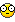## Recommended Posts

From an old post I was trying some "skinning" of my control.

(Posts: gafrost and Larry @ http://www.autoitscript.com/forum/index.ph...st&p=86632)

I made a simple BMP to test with: 300x200.bmp

And no luck.

But, by debugging skilles are not yet up to the task of finding the problem.

So I could use a little help here I think to better understand what is going wrong.

Here is that code:

```#include <GuiConstants.au3>

\$file = ".\300x200.bmp"
\$bmpwidth = 300
\$bmpheight = 200
\$transparentcolor = 0xffffff

\$my_gui = GUICreate("   SkinIt", \$bmpwidth +10, \$bmpheight + 10 ,-1,-1,\$WS_POPUP)
GUICtrlCreatePic(\$file, 0, 0, \$bmpwidth, \$bmpheight )

;_SkinMyGUI(\$my_gui,\$file,\$transparentcolor ) ;; uncomment for use  <<<<

GUISetState()

While 1
\$msg = GUIGetMsg()
Select
Case \$msg = \$GUI_EVENT_CLOSE
ExitLoop
Case Else
;;;
EndSelect
WEnd
Exit

Func _SkinMyGUI(\$hwnd, \$cPicture, \$cTransparent)
Dim \$ret, \$hinstance, \$hObj
Dim \$GWL_HINSTANCE = -6
Dim \$IMAGE_BITMAP = 0

Dim \$hRgn, \$tRgn, \$obj
Dim \$X, \$Y, \$X0
Dim \$hDC, \$BM
Dim \$BM
Dim \$bmType = 1
Dim \$bmWidth = 2
Dim \$bmHeight = 3
Dim \$bmWidthBytes = 4
Dim \$bmPlanes = 5
Dim \$bmBitsPixel = 6
Dim \$bmBits = 7

\$ret = DllCall("user32.dll","int","GetWindowLong","hWnd",\$hwnd, "int",\$GWL_HINSTANCE)
\$hinstance = \$ret

\$cPicture = \$ret

\$BM = DllStructCreate ("int;int;int;int;int;int;int")
If @error Then
MsgBox(0, "", "Error in DllStructCreate " & @error);
Exit
EndIf

\$hDC = DllCall("gdi32.dll", "int", "CreateCompatibleDC", "int", 0)
If \$hDC Then
DllCall("gdi32.dll", "hwnd", "SelectObject", "int", \$hDC, "hwnd", \$cPicture)
DllCall("gdi32.dll", "hwnd", "GetObject", "hwnd", \$cPicture, "int", DllStructGetSize (\$BM), "ptr", DllStructGetPtr (\$BM))
\$hRgn = DllCall("gdi32.dll", "hwnd", "CreateRectRgn", "int", 0, "int", 0, "int", DllStructGetData (\$BM, \$bmWidth), "int", DllStructGetData (\$BM, \$bmHeight))
For \$Y = 0 To DllStructGetData (\$BM, \$bmHeight)
For \$X = 0 To DllStructGetData (\$BM, \$bmWidth)
While \$X <= DllStructGetData (\$BM, \$bmWidth) And _GetPixel(\$hDC, \$X, \$Y) <> \$cTransparent
\$X = \$X + 1
WEnd
\$X0 = \$X
While \$X <= DllStructGetData (\$BM, \$bmWidth) And _GetPixel(\$hDC, \$X, \$Y) = \$cTransparent
\$X = \$X + 1
WEnd
If \$X0 < \$X Then
\$tRgn = DllCall("gdi32.dll", "hwnd", "CreateRectRgn", "int", \$X0, "int", \$Y, "int", \$X, "int", \$Y + 1)
DllCall("gdi32.dll", "int", "CombineRgn", "hwnd", \$hRgn, "hwnd", \$hRgn, "hwnd", \$tRgn, "int", 4)
DllCall("gdi32.dll", "int", "DeleteObject", "hwnd", \$tRgn)
EndIf
Next
Next
\$obj = DllCall("gdi32.dll", "hwnd", "SelectObject", "int", \$hDC, "hwnd", \$cPicture)
DllCall("gdi32.dll", "int", "DeleteObject", "hwnd", \$obj)
EndIf
DllCall("gdi32.dll", "int", "DeleteDC", "hwnd", \$hDC)
DllStructDelete (\$BM)
DllCall("user32.dll", "hwnd", "SetWindowRgn", "hwnd", \$hwnd, "hwnd", \$hRgn, "int", 1)
EndFunc;==>_GetBitmapRegion

Func _GetPixel(\$hDC, \$X, \$Y)
Dim \$c
\$c = DllCall("gdi32.dll", "int", "GetPixel", "int", \$hDC, "int", \$X, "int", \$Y)
Return \$c
EndFunc;==>_GetPixel```

Thanks,

gsb

"Did you ever stop to think? ...and forget to restart!"

##### Share on other sites

Ok.

Figured that out.

TYVMgsb

"Did you ever stop to think? ...and forget to restart!"

##### Share on other sites

EDIT: Nevermind. I am an idiot.

NOTE TO SELF: Read instructions carefully.

Edited by this-is-me

Who else would I be?

## Create an account

Register a new account

• ### Recently Browsing   0 members

×

• Wiki

• Back

• #### Beta

• Git
• FAQ
• Our Picks
×
• Create New...